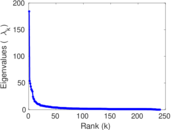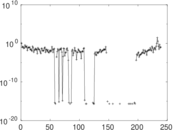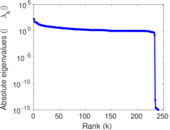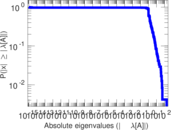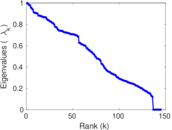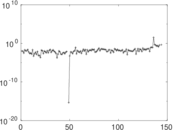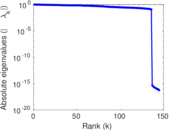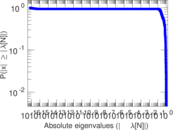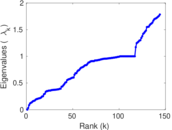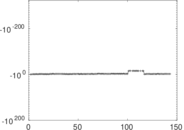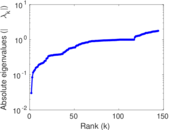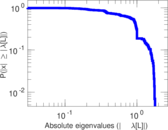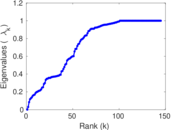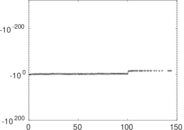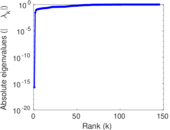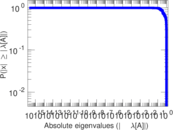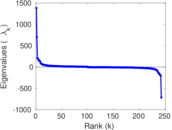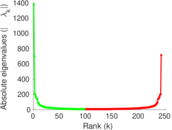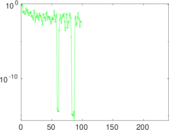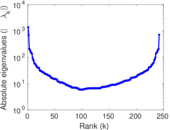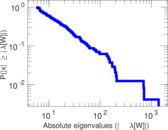# Wikiquote edits (eu)

This is the bipartite edit network of the Basque Wikiquote. It contains users and pages from the Basque Wikiquote, connected by edit events. Each edge represents an edit. The dataset includes the timestamp of each edit.

 Code `qeu` Internal name `edit-euwikiquote` Name Wikiquote edits (eu) Data source http://dumps.wikimedia.org/ AvailabilityDataset is available for download Consistency checkDataset passed all tests Category Authorship network Dataset timestamp 2017-10-20 Node meaning User, article Edge meaning Edit Network formatBipartite, undirected Edge typeUnweighted, multiple edges Temporal dataEdges are annotated with timestamps

## Statistics

 Size n = 1,380 Left size n1 = 242 Right size n2 = 1,138 Volume m = 5,591 Unique edge count m̿ = 2,604 Wedge count s = 161,015 Claw count z = 11,478,179 Cross count x = 766,536,575 Square count q = 66,924 4-Tour count T4 = 1,187,072 Maximum degree dmax = 1,627 Maximum left degree d1max = 1,627 Maximum right degree d2max = 184 Average degree d = 8.102 90 Average left degree d1 = 23.103 3 Average right degree d2 = 4.913 01 Fill p = 0.009 455 48 Average edge multiplicity m̃ = 2.147 08 Size of LCC N = 1,113 Diameter δ = 11 50-Percentile effective diameter δ0.5 = 3.454 13 90-Percentile effective diameter δ0.9 = 5.588 82 Median distance δM = 4 Mean distance δm = 4.006 22 Gini coefficient G = 0.784 428 Balanced inequality ratio P = 0.182 257 Left balanced inequality ratio P1 = 0.117 868 Right balanced inequality ratio P2 = 0.234 842 Relative edge distribution entropy Her = 0.820 699 Power law exponent γ = 2.777 57 Tail power law exponent γt = 2.011 00 Tail power law exponent with p γ3 = 2.011 00 p-value p = 0.000 00 Left tail power law exponent with p γ3,1 = 1.701 00 Left p-value p1 = 0.230 000 Right tail power law exponent with p γ3,2 = 6.761 00 Right p-value p2 = 0.239 000 Degree assortativity ρ = −0.131 391 Degree assortativity p-value pρ = 1.687 75 × 10−11 Spectral norm α = 184.230 Algebraic connectivity a = 0.030 187 7 Spectral separation |λ1[A] / λ2[A]| = 3.427 63 Controllability C = 908 Relative controllability Cr = 0.660 844

## Plots

### Fruchterman–Reingold graph drawing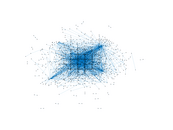### Degree distribution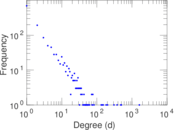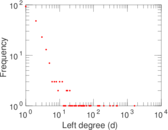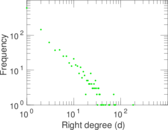### Cumulative degree distribution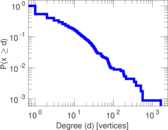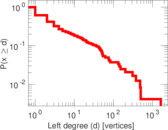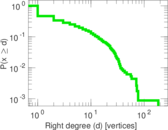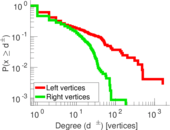### Lorenz curve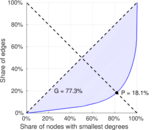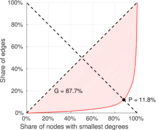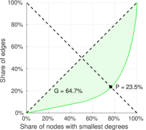### Spectral distribution of the adjacency matrix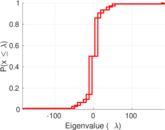### Spectral distribution of the normalized adjacency matrix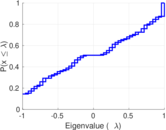### Spectral distribution of the Laplacian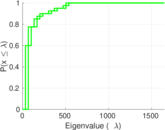### Spectral graph drawing based on the adjacency matrix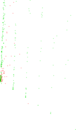### Spectral graph drawing based on the Laplacian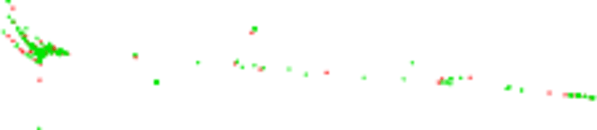### Spectral graph drawing based on the normalized adjacency matrix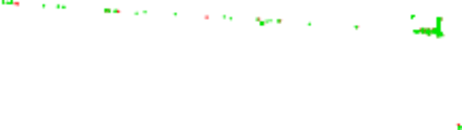### Degree assortativity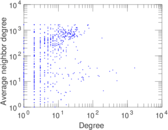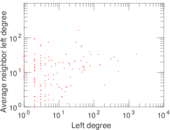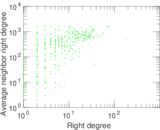### Zipf plot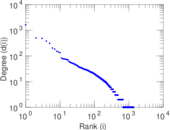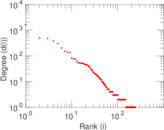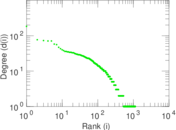### Hop distribution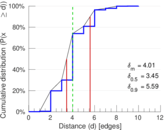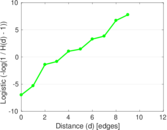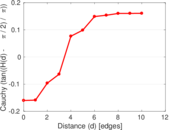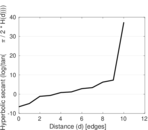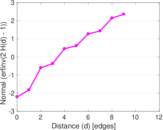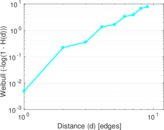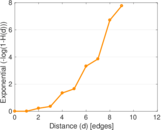### Double Laplacian graph drawing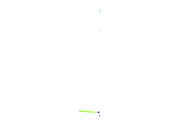### Delaunay graph drawing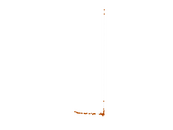### Edge weight/multiplicity distribution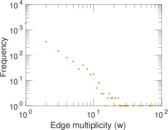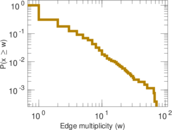### Temporal distribution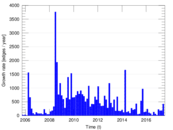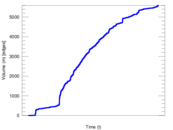### Temporal hop distribution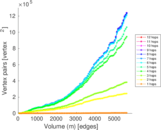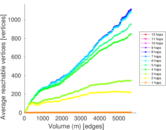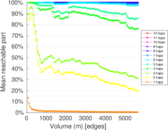### Diameter/density evolution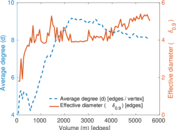### Matrix decompositions plots